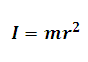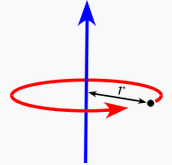# Moment of Inertia of a Point Mass Calculator

This Calctown Calculator calculates the Moment of Interia of a Point Mass about its axis of rotation.

kg
m

#### Result

kg-m2Click here to view image

where

I = Moment of Inertia

m = given point mass

r = distance of point mass from its axis of rotation